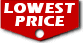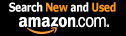# Ordinary Differential Equations (Dover Books on Mathematics) / Tenenbaum, Morris

List Price: \$28.95
Our Price: \$25.49For Bulk orders
Quantity

Used Book Price:
/ Tenenbaum, Morris
Publisher: Dover Publications
Availability:In Stock.
Sales Rank: 24283
 ISBN-10: 0486649407ISBN-13: 9780486649405

Similar Books
 Partial Differential Equations for Scientists and Engineers (Dover Books on Mathematics) Linear Algebra (Dover Books on Mathematics) Fourier Series (Dover Books on Mathematics) Schaum's Outline of Differential Equations, 4th Edition (Schaum's Outlines) Differential Equations (Quick Study Academic) Calculus: An Intuitive and Physical Approach (Second Edition) (Dover Books on Mathematics) Differential Equations For Dummies Probability Theory: A Concise Course (Dover Books on Mathematics) An Introduction to Information Theory: Symbols, Signals and Noise (Dover Books on Mathematics) Introduction to Topology: Third Edition (Dover Books on Mathematics)

This unusually well-written, skillfully organized introductory text provides an exhaustive survey of ordinary differential equations � equations which express the relationship between variables and their derivatives. In a disarmingly simple, step-by-step style that never sacrifices mathematical rigor, the authors � Morris Tenenbaum of Cornell University, and Harry Pollard of Purdue University � introduce and explain complex, critically-important concepts to undergraduate students of mathematics, engineering and the sciences.
The book begins with a section that examines the origin of differential equations, defines basic terms and outlines the general solution of a differential equation-the solution that actually contains every solution of such an equation. Subsequent sections deal with such subjects as: integrating factors; dilution and accretion problems; the algebra of complex numbers; the linearization of first order systems; Laplace Transforms; Newton's Interpolation Formulas; and Picard's Method of Successive Approximations.
The book contains two exceptional chapters: one on series methods of solving differential equations, the second on numerical methods of solving differential equations. The first includes a discussion of the Legendre Differential Equation, Legendre Functions, Legendre Polynomials, the Bessel Differential Equation, and the Laguerre Differential Equation. Throughout the book, every term is clearly defined and every theorem lucidly and thoroughly analyzed, and there is an admirable balance between the theory of differential equations and their application. An abundance of solved problems and practice exercises enhances the value of Ordinary Differential Equations as a classroom text for undergraduate students and teaching professionals. The book concludes with an in-depth examination of existence and uniqueness theorems about a variety of differential equations, as well as an introduction to the theory of determinants and theorems about Wronskians.

Now you can buy Books online in USA,UK, India and more than 100 countries.
*Terms and Conditions apply
Disclaimer: All product data on this page belongs to.
No guarantees are made as to accuracy of prices and information.